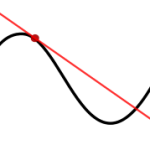# General Solution of Differential Equation

## How to Find the General Solution of Differential EquationProblems with differential equations are asking you to find an unknown function or functions, rather than a number or set of numbers as you would normally find with an equation like f(x) = x2 + 9.

For example, the differential equation dydx = 10x is asking you to find the derivative of some unknown function y that is equal to 10x.

## General Solution of Differential Equation: Example

Example problem #1: Find the general solution for the differential equation dydx = 2x.

Step 1: Use algebra to get the equation into a more familiar form for integration:
dydx = 2x →
dy = 2x dx

Step 2: Integrate both sides of the equation:
∫dy = ∫2x dx →
&int1 dy = &int2x dx →
y = x2 + C

Example problem #2: Find the general solution for the differential equation dydx = x2 – 3

Step 1: Use algebra to get the equation into a more familiar form for integration:
dydx = x2 – 3→
dy = x2 – 3 dx

Step 2: Integrate both sides of the equation:
∫ dy = ∫x2 – 3 dx →
∫ 1 dy = ∫x2 – 3 dx →
y = x33 -3x + C

Sample problem #3: Find the general solution for the differential equation θ2 dθ = sin(t + 0.2) dt.

Step 1: Integrate both sides of the equation:
∫ θ2 dθ = ∫sin(t + 0.2) dt →
θ3 = -cos(t + 0.2) + C
That’s how to find the general solution of differential equations!

Tip: If your differential equation has a constraint, then what you need to find is a particular solution. For example, dydx = 2x ; y(0) = 3 is an initial value problem that requires you to find a solution that satisfies the constraint y(0) = 3.

## References

Larson & Edwards. Calculus.

CITE THIS AS:
Stephanie Glen. "General Solution of Differential Equation" From StatisticsHowTo.com: Elementary Statistics for the rest of us! https://www.statisticshowto.com/differential-equations/general-solution-of-differential-equation/